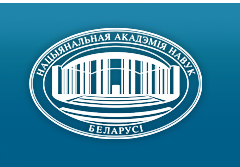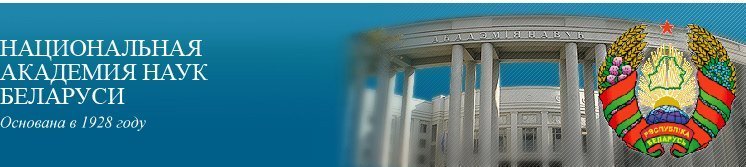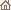Главная Новости Об академии Деятельность академии Члены академии Организации Издания академии Мероприятия Контакты Электронные обращения Справочная информация Карта сайта

# Известия Академии наук Беларуси. Сер. физ.-матем. наук, 1997, No.3/ Издания академии / Научные журналы

Известия Академии наук Беларуси. Сер. физ.-матем. наук, 1997, No.3Известия Академии наук Беларуси СЕРИЯ ФИЗИКО-МАТЕМАТИЧЕСКИХ НАУКИздатель: Беларуская навука, Минск, Республика Беларусь

### Номер 3, 1997## СОДЕРЖАНИЕ

### МАТЕМАТИКА

Bernik V.I., Borbat V.I.
The polynomials with overfalls of the values of coefficients and A. Baker's conjecture. pp. 5--8

Summary: It is proved that, for any e > 0, the inequality |P(x)| < (Õi=14 max (| ai |, l))-1-e has an infinite set of its solutions in polynomials with integer coefficients only for a zero measure set. Thus, this gives the confirmation of A. Baker's conjecture (1966) for n = 4.

Malyutin V.B.
Approximations of functional integrals generated by a differential equation with the coefficients from a commutative Banach superalgebra. pp. 9--13

Summary: Approximations of functional integrals generated by a differential equation with the coefficients from a commutative Banach superalgebra are considered.

Pekarski A.A.
Inequalities for the derivatives of rational functions in the Lorentz spaces. pp. 14--16

Summary: We give some new inequalities for the derivatives of rational functions, which are related to the results of E.A. Sevastyanov, H. Stahl and the author.

Medvedev G.A.
A software reliability model using the computing process features. pp. 17--21

Summary: Some additional information about computer programs is suggested to use for forming a software reliability model. This approach is examined by means of simulation of nine testing processes.

Zhuk Ye.Ye., Harin Yu.S.
Asymptotic estimating the risk for classification of dependent observations. pp. 22--27

Summary: An optimal decision rule, which has minimal risk (classification error probability), is constructed under dependence of the class numbers. Risk asymptotic expressions are obtained under increasing size of classified samples. The case of the Markov class number dependence is investigated.

Yudin M.D.
Applications of generalized Kolmogorov's relation to sums of dependent vectors. pp. 28--31

Summary: The limit distributions of sums of dependent random vectors under the normal and the Poisson conditions are investigated.

Lazakovich N.V., Stashulenok A.P.
Associated in the Ito sense solutions of differential equations in the direct product of algebras of generalized stochastic processes. pp. 32--38

Summary: Some approximation problems for many-dimensional stochastic integrals and Ito's differential equations in the direct product of algebras of generalized random processes are considered.

Isakova G.A.
Some properties of covariation of complex stable random variables. pp. 39--43

Summary: A notion of covariation of complex symmetric stable random variables is introduced and its properties are established.

Lukashevich N.A., Chichurin A.V.
On existence of the surfaces that are generated by nonlinear differential equations of the second order. pp. 44--47

Summary: Conditions that guarantee the existence of regular coefficients of the first quadratic form, which is defined by differential equation d2w / d v2 = (A0 (w, v))-1 ( A1 (w, v) (dw/dv)3 + A2 (w, v) (dw/dv)2 + A3 (w, v) dw/dv + A4(w, v) ), where Aj(w, v) (j = 1,4) are continuously differentiable functions of w, v and A0(w, v) is doubly continuously differentiable function of w, v in a domain, are obtained.

Benedictovich V.I.
Minmax length triangulations and the local optimization procedure. pp. 48--50

Summary: A minmax length triangulation M(V) of a point set V is a triangulation that minimizes the length of its longest edge among all triangulations of V. The Delauney triangulation D(V) and the greedy triangulation G(V) are investigated for the optimality. It is shown that 2/Ö3 is the worst possible ratio for the Delaunay triangulation and that the procedure of local optimization does not give the optimum.

Kremen' Ye.V.
Multiple orthogonal difference sweep method for three-point grid equations of the general form. pp. 51--56

Summary: A new algorithm of the multiple orthogonal difference pivotal consideration based on the well-known S.K. Godunov method for the three-point grid equations with the separated two-point conditions is developed. The method is conceptually simple and convenient for numerical realization.

Safonov V.G.
On critical multiply local formations of finite groups. pp. 57--61

Summary: We give a description of soluble minimal n-multiply local non-h-formations, where h is some m-multiply local formation, m > n > 0.

Gladyshev M.T.
On theory of the Khohlov -- Zabolotskaya equation. pp. 62--67

Summary: Problems of deriving the Khohlov -- Zabolotskaya equation, a formulation of the boundary problem, investigation of the linear approximation as well as investigation of the group properties and particular solutions are considered.

### ФИЗИКА

Zhur T.A., Nemanova I.T., Ryabushko A.P.
Relativistic equations for translational movement of a rotating particle in the medium. pp. 68--70

Summary: A system of differential equations, which describes the relativistic translation of a rotating particle in the internal gravitational field induced by the gravity center contained in a gas-powder ball. is introduced. The system was derived on the basis of Papapetrou's equations with some additional conditions on Sa b : S0i = K Sij uj.

Hoang Quoc Khanh, Le Van Hoang, Komarov L.I.
Convergence of the operator method and the free constant choice problem. pp. 71--75

Summary: Convergence of the operator method is investigated for the problem of quantum anharmonic oscillator. We show that the iterative process of the operator method is convergent for the entire range of the coupling constant and for an arbitrary choice of the free constant. A method for the choice of the free constant such that the iterative process of the operator method converges most rapidly is offered.

Mityanok V.V.
On the problem of simulation of cefeides with pulsatory stars. pp. 76--81

Summary: Numerical solutions to the equation of star's pulsations are obtained. The character of pulsations depending on the initial state are studied.

Suchvalo S.V., Vlasov V.V.
Orientation effects in cubic magnets. pp. 82--85

Summary: Theoretical study of the spin-reorientation transformation in cubic magnets are reported.

Levchenko V.I., Postnova L.I.
Controllable formation of the concentration, gradients and p-n-junctions in epitaxial PbS films. pp. 86--89

Summary: Kinetics of changing the electrophysical parameters of the heteroepitaxial PbS films on BaF2 during the vacuum heat treatment is studied experimentally. It is shown that vacuum annealing may be used as an effective method for creation of intrinsic unstoichiometric defects concentration gradients and p-n-junctions.

Markevich A.V., Sokolovski T.D.
Lattice dynamics and elastic moduli of ordered alloy NiCo. pp. 90--92

Summary: The bond energy calculations of the atoms of nickel and cobalt, calculations of dispersion relations, mean-square dynamical displacement and the mean-square velocities of the atoms of nickel and cobalt in ordered alloy NiCo are carried out.

Yakimovich V.N., Konstantinov V.I., Orlik L.K.
The magneto-optical Q-factor of Cd1-xMnxTe crystals. pp. 93--95

Summary: The dispersion of absorption coefficient and the Verde constant of Cd1-xMnxTe crystals is investigated. Their magneto-optical Q-factor is determined. The dispersive curves of the magneto-optical Q-factor have a well-defined maximum near the edge absorption of Cd1-xMnxTe crystal.

Petrashko V.V., Semenenko Yu.A., Novysh B.V., Zhygadlo N.D., Turtsevich D. M.
Preparing and properties of superconducting ceramics Bi(Pb)-2223 with Os and Ag2O supplements. pp. 96--100

Summary: Influence of osmium and Ag2O additions on superconducting properties of Bi(Pb)-2223 compound is investigated. Small Os and AgO additions were found out to improve the transport properties of the materials under certain synthesis conditions.

Myasoedov A.V., Syrtsov S.R.
Effect of sintering superconducting ceramics Ba0.9Pb0.1TiO3 on the positive temperature coefficient of resistance devices. pp. 101--102

Summary: The positive temperature coefficient of resistivity is investigated for 0.5 atom%-Y-doped Ba0.9Pb0.1TiO3 ceramics. The room-temperature resistivity decreases when the sintering time increasing. Effect of sintering time varying from 3 to 30 minutes at different sintering temperature on the PTCR is studied.

Boltushkin V.I., Goltsev V.P., Goltsev M.V., Chaevski V.V.
Influence of the composition of triple nitrides on hardening vacuum-plasma coatings. pp. 103--105

Summary: The structure and phase composition of triple nitrides Ti--Zr--N, Ti--Cr--N, Zr--Cr--N and Zr--Mo--N is investigated. Dependencies of the microhardness and crystal lattice parameters of these systems on their compositions are studied.

Kvasov N.T., Kuznechik O.O., Sologub L.V.
Acoustic relaxation of the crystal lattice under ion irradiation. pp. 106--108

Summary: Acoustic relaxation of the crystal lattice of silicon when an atom being knocked out is analyzed. It is shown that an elastic N-wave is generated in the crystal. The wave interacts with the initial defects and causes a modification of their geometric location and the thermodynamic state. Besides, new defects in the field of elastic oscillations may be also generated.

Brinkevich D.I., Vabishchevich S.A., Petrov V.V.
Influence of germanium on the impurity composition in silicon single crystal. pp. 109--112

Summary: Influence of the Ge isovalent impurity on the impurity composition of the Czochralski silicon is investigated by means of the Hall effect and conductivity measurements methods. It is established that Ge changes the relation between the interstitial and substitution components of the boron impurity and increases boron concentration in the electrically active site. Presence of Ge in the melt reduces the phosphorus segregation coefficient. Possible mechanisms of the impurities behavior are discussed.

Boltushkin A.V., Shadrov V.G., O'Grady C., Cambridge D., Tagirov R.I., Arhipenko Zh.N.
Study of magnet granular interaction in electrodeposited Co-Ni-W films. pp. 113--116

Summary: The results of interaction effects study in electrodeposited Co--Ni--W films through remanence and dM curves are reported.

Metel'sky G.L.
Split line graphs of hypergraphs having a bounded rank. pp. 117--122

Summary: It is known that, given any integer r <>³ 3, both Lr and Lrl (the class of line graphs of r-uniform hypergraphs and the class of line graphs of linear r-uniform hypergraphs, respectively) cannot be characterized by finite lists of forbidden induced subgraphs. In this paper the existence of such characterizations of these classes is shown provided the graphs under consideration are split graphs. The corresponding lists for Lr and Lrl are given.

Sadyhov R.H., Samohval V.A.
Identification of signatures on the base of machinery of discriminant functions. pp. 123--126

Summary: The problem of handwritten signature verification is studied, using a simplified version of synthetic discriminant function. The 2-D BIFORE transform is applied to the data compression and feature extraction, resulting in a reliable verification.

Minchenko L.I., Sakolchik P.P.
Epiderivatives of marginal functions in nonlinear programming. pp. 127--131

Summary: Marginal functions in nonlinear programming under Janin's constant rank regularity condition is considered. In particular, epidifferentiability and directional differentiability of marginal functions are proved.

### КРАТКИЕ СООБЩЕНИЯ

Tsegelnik V.V.
On two classes of exact self-similar solutions of Boussinesq's system. pp. 132--133

Summary: For the classical Boussinesq equation representable as a system of nonlinear partial differential equations, formulae for exact self-similar solutions, which are expressed in terms of solutions to the 2nd and the 4th Painleve equations, are obtained.

Misevich V.S.
An addition to the paper "Asymmetric aberration experiment with the result predetermined by Bradly's experiment". pp. 134--135

Summary: It is shown that measurement results of the asymmetric aberration experiment with a telescope and diffraction grating, which was obtained before, must be interpreted with the help of a system of two equations in two unknowns. This system can be solved numerically.

### АННОТАЦИИ ДЕПОНИРОВАННЫХ СТАТЕЙ

Zverovich I.E.
Application of generalized Krausz's theorem to the decision problem for the line graphs of the line 3-uniform hypergraphs. p. 136

Prusov V.I.
Oscillations of the strip under the stamp action. pp. 136--137

Minyuk S.A., Lomakina L.V.
On controllability and completeness of one-parameter discrete systems. p. 137/ Издания академии / Научные журналы / К началу страницы

Разработана и поддерживается Николаем Н. Костюковичем. Последнее обновление: 7 ноября 2006 г.
Создана при участии Игнатия И. Корсака
Копирайт © 1998-2006 Национальная академии наук Беларуси
Копирайт © 1997 Издательство "Беларуская навука"